Home Practice
For learners and parents For teachers and schools
Textbooks
Full catalogue
Pricing SupportLog in

We think you are located in United States. Is this correct?

# 14.4 Union and intersection

## 14.4 Union and intersection (EMA7Z)

temp text
Union

The union of two sets is a new set that contains all of the elements that are in at least one of the two sets. The union is written as $$A \cup B$$ or “$$A \text{ or } B$$”.

Intersection

The intersection of two sets is a new set that contains all of the elements that are in both sets. The intersection is written as $$A \cap B$$ or “$$A \text{ and } B$$”.

The figure below shows the union and intersection for different configurations of two events in a sample space, using Venn diagrams.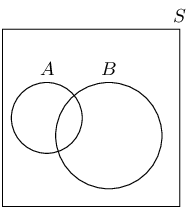Figure 14.1: The unions and intersections of different events. Note that in the middle column the intersection, $$A \cap B$$, is empty since the two sets do not overlap. In the final column the union, $$A \cup B$$, is equal to $$A$$ and the intersection, $$A \cap B$$, is equal to $$B$$ since $$B$$ is fully contained in $$A$$.
Textbook Exercise 14.4

A group of learners are given the following Venn diagram: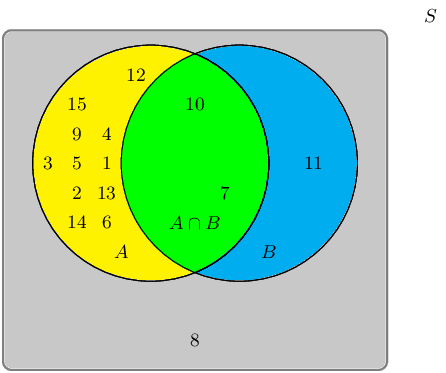The sample space can be described as $$\{ n:n \text{ } \epsilon \text{ } \mathbb{Z}, \text{ } 1 \leq n \leq 15 \}$$.

They are asked to identify the event set of the intersection between event set $$A$$ and event set $$B$$, also written as $$A \cap B$$. They get stuck, and you offer to help them find it.

Which set best describes the event set of $$A \cap B$$?

• $$\{7;10;11\}$$
• $$\{1;2;3;4;5;6;7;9;10;11\}$$
• $$\{1;2;3;4;5;6;7;9;10\}$$
• $$\{7;10\}$$

The intersection between event set $$A$$ and event set $$B$$, also written as $$A \cap B$$, can be shaded as follows: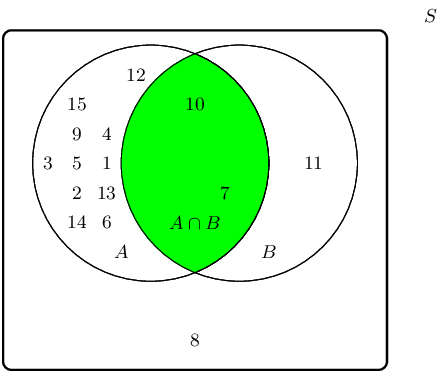Therefore the event set $$\{7;10\}$$ best describes the event set of $$A \cap B$$.

A group of learners are given the following Venn diagram: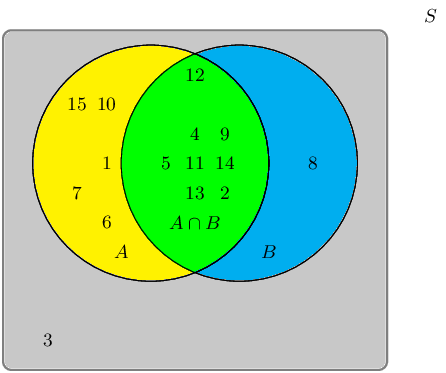The sample space can be described as $$\{ n:n \text{ } \epsilon \text{ } \mathbb{Z}, \text{ } 1 \leq n \leq 15 \}$$

They are asked to identify the event set of the union between event set $$A$$ and event set $$B$$, also written as $$A \cup B$$. They get stuck, and you offer to help them find it.

Which set best describes the event set of $$A \cup B$$?

• $$\{1;6;7;10;15\}$$
• $$\{1;2;4;5;6;7;8;9;10;11;12;13;14;15\}$$
• $$\{2;4;5;9;10;11;12;13;14\}$$
• $$\{3\}$$

The union between event set $$A$$ and event set $$B$$, also written as $$A \cup B$$, can be shaded as follows: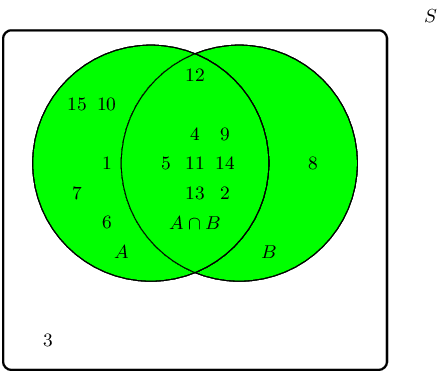Therefore the event set $$\{1;2;4;5;6;7;8;9;10;11;12;13;14;15\}$$ best describes the event set of $$A \cup B$$.